Checkout JEE MAINS 2022 Question Paper Analysis : Checkout JEE MAINS 2022 Question Paper Analysis :

# Area of a Circle

Area of a circle is the region occupied by the circle in a two-dimensional plane. It can be determined easily using a formula, A = πr2(Pi r-squared) where r is the radius of the circle. The unit of area is the square unit, such as m2, cm2, etc.

 Area of Circle = πr2 or πd2/4, square units where π = 22/7 or 3.14

The area of the circle formula is useful for measuring the space occupied by a circular field or a plot. Suppose you have the plot to fence it, then the area formula will help you to check how much fencing is required. Or suppose you have to buy a table cloth, then how many portions of cloth is needed to cover it completely.

Hence, the concept of area as well as the perimeter is introduced in Maths, to figure out such scenarios. But, one common question that arises among most people is “does a circle have volume?”. The answer is “No”. Since a circle is a two-dimensional shape, it does not have volume. It has only an area and perimeter. So, we don’t have the volume of a circle. In this article, let us discuss in detail the area of a circle, surface area and its circumference with examples.

## What is a Circle?

A circle closed plane geometric shape. In technical terms, a circle is a locus of a point moving around a fixed point at a fixed distance away from the point. Basically, a circle is a closed curve with its outer line equidistant from the center. The fixed distance from the point is the radius of the circle. In real life, you will get many examples of the circle such as a wheel, pizzas, a circular ground, etc. Now let us learn, what are the terms used in the case of a circle.

The radius of the circle is the line that joins the center of the circle to the outer boundary. It is usually represented by ‘r’ or ‘R’. In the formula for the area and circumference of a circle, radius plays an important role which you will learn later.

Diameter

The diameter of the circle is the line that divides the circle into two equal parts. In an easy way we can say, it is just the double of the radius of the circle and is represented by ‘d’ or ‘D’. Therefore,

d = 2r or D = 2R

If the diameter of the circle is known to us, we can calculate the radius of the circle, such as;

r = d/2 or R = D/2

Circumference of Circle

A perimeter of closed figures is defined as the length of its boundary. When it comes to circles, the perimeter is given using a different name. It is called the “Circumference” of the circle. This circumference is the length of the boundary of the circle. If we open the circle to form a straight line, then the length of the straight line is the circumference. To define the circumference of the circle, knowledge of a term known as ‘pi’ is required. Consider the circle shown in the fig. 1, with centre at O and radius r.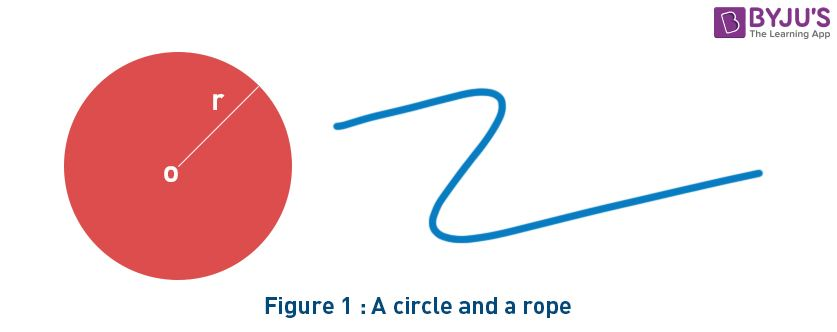The perimeter of the circle is equal to the length of its boundary. The length of rope which wraps around its boundary perfectly will be equal to its circumference, which can be measured by using the formula:

Circumference / Perimeter = 2πr units

where r is the radius of the circle.

π, read as ‘pi’ is defined as the ratio of the circumference of a circle to its diameter. This ratio is the same for every circle. Consider a circle with radius ‘r’ and circumference ‘C’. For this circle

• π = Circumference/Diameter
• π = C/2r
• C = 2πr

The same is shown in fig. 2.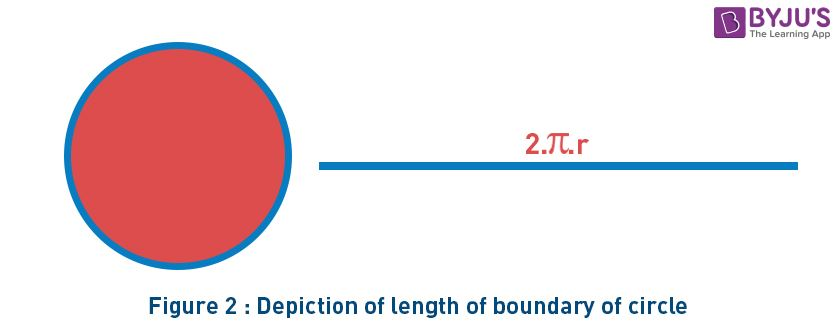## What is Area of Circle?

Area of a circle is the region covered or enclosed within its boundary. It is measured in square units.

Any geometrical shape has its own area. This area is the region that occupies the shape in a two-dimensional plane. Now we will learn about the area of the circle. So the area covered by one complete cycle of the radius of the circle on a two-dimensional plane is the area of that circle. Now how can we calculate the area for any circular object or space? In this case, we use the formula for the circle’s area. Let us discuss the formula now.

## Area of a Circle Formula

Let us take a circle with radius r.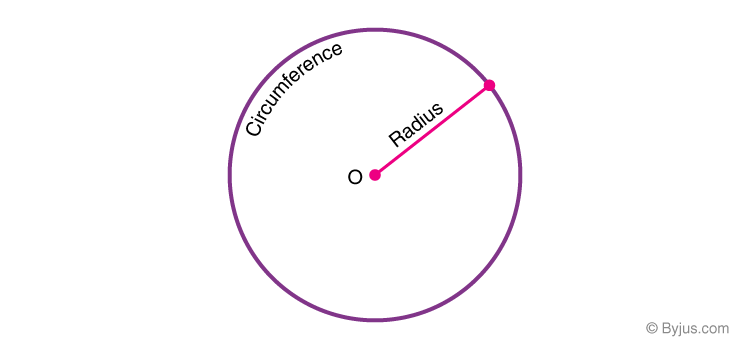In the above figure, we can see a circle, where radius r from the center ‘o’ to the boundary of the circle. Then the area for this circle, A, is equal to the product of pi and square of the radius. It is given by;

 Area of a Circle, A = πr2 square units

Here, the value of pi, π = 22/7 or 3.14 and r is the radius.

## Derivation of Area of Circle

Area of a circle can be visualized & proved using two methods, namely

• Determining the circle’s area using rectangles
• Determining the circle’s area using triangles

Let us understand both the methods one-by-one.

### Using Areas of Rectangles

The circle is divided into 16 equal sectors, and the sectors are arranged as shown in fig. 3. The area of the circle will be equal to that of the parallelogram-shaped figure formed by the sectors cut out from the circle. Since the sectors have equal area, each sector will have an equal arc length. The red coloured sectors will contribute to half of the circumference, and blue coloured sectors will contribute to the other half. If the number of sectors cut from the circle is increased, the parallelogram will eventually look like a rectangle with length equal to πr and breadth equal to r.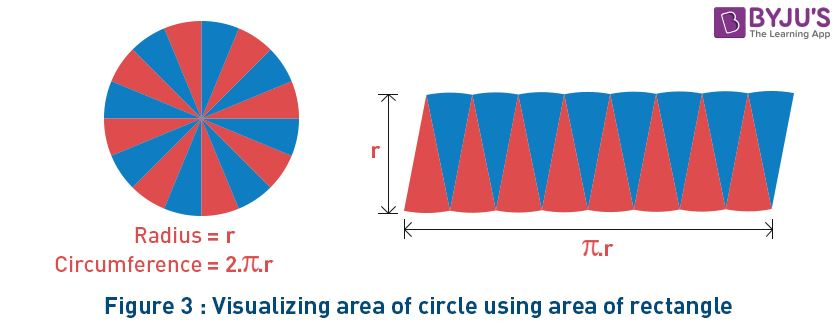The area of a rectangle (A) will also be the area of a circle. So, we have

• A = π×r×r
• = πr2

### Using Areas of  Triangles

Fill the circle with radius r with concentric circles. After cutting the circle along the indicated line in fig. 4 and spreading the lines, the result will be a triangle. The base of the triangle will be equal to the circumference of the circle, and its height will be equal to the radius of the circle.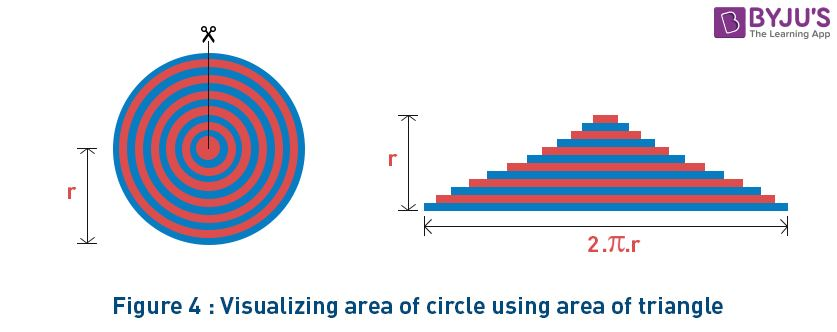So, the area of the triangle (A) will be equal to the area of the circle. We have

A = 1/2×base×height

A = 1/2×(2πr)×r

A = πr2

## Surface Area of Circle

A circle is nothing but the 2-D representation of a sphere. The total area that is taken inside the boundary of the circle is the surface area of the circle. When we say we want the area of the circle, then we mean the surface area of the circle itself. Sometimes, the volume of a circle also defines the area of a circle.

When the length of the radius or diameter or even the circumference of the circle is already given, then we can use the surface formula to find out the surface area. The surface is represented in square units.

The surface area of the circle = A =  π x r2

## How to Find Area of a Circle?

As we know, the area of circle is equal to pi times square of its radius, i.e. π x r2. To find the area of circle we have to know the radius or diameter of the circle.
For example, if the radius of circle is 7cm, then its area will be:
Area of circle with 7 cm radius = πr2 = π(7)2 = 22/7 x 7 x 7 = 22 x 7 = 154 sq.cm.

Also, if we know the circumference of the circle, then we can find the area of circle.
How?
Since, the circumference is 2 times of product of pi and radius of circle, such as:
C = 2πr
Therefore, here we can find the value of radius,
r = C/2π
Once, we have evaluated the value of radius, we can easily find the area.

## Difference Between Square Area and Circle Area

The area of a circle is estimated to be 80% of area of square, when the diameter of the circle and length of side of the square is the same.

Students can also do an activity by inserting a circular object into a square shape with same diameter and side-length, respectively.

If area of square is 100 sq.unit, then the area of circle will be approximately 80 sq.unit of it.

## Video Lesson

### Area of a Circle## Solved Examples on Area of a Circle

We have discussed till now the different parameters of the circle such as area, perimeter or circumference, radius and diameter. Let us solve some problems based on these formulas to understand the concept of area and perimeter in a better way.

Example 1:

What is the radius of the circle whose surface area is 314.159 sq.cm?

Solution:

By the formula of the surface area of the circle, we know;

A = π x r2

Now, substituting the value:

314.159 = π x r2

314.159 = 3.14 x r2

r2 = 314.159/3.14

r2 = 100.05

r = √100.05

r = 10 cm

Example 2:

Find the circumference and the area of circle if the radius is 7 cm.

Solution:

Given: Radius, r = 7 cm

We know that the circumference/ perimeter of the circle is 2πr cm.

Now, substitute the radius value, we get

C = 2  × (22/7)× 7

C = 2×22

C = 44 cm

Thus, the circumference of the circle is 44 cm.

Now, the area of the circle is πrcm2

A = (22/7) × 7 × 7

A = 22 × 7

A = 154 cm

Example 3:

If the longest chord of a circle is 12 cm, then find the area of circle.

Solution:

Given that the longest chord of a circle is 12 cm.

We know that the longest chord of a circle is the diameter.

Hence, d = 12 cm.

So, r = d/2 = 12/2 = 6 cm.

The formula to calculate the area of circle is given by,

A = πrsquare units.

Now, substitute r = 6 cm in the formula, we get

A = (22/7)×6×6 cm2

A = (22/7)×36 cm2

A = 792/7 cm2

A = 113.14 cm(Rouned to 2 decimal places)

Therefore the area of circle is 113.14 cm2.

## Frequently Asked Questions on Area of Circle

### What is meant by area of circle?

The area of circle is the region occupied by circle in the two-dimensional space.

### How to calculate the area of a circle?

The area of circle can be calculated by using the formulas:
Area = π x r2, in terms of radius ‘r’.
Area = (π/4) x d2, in terms of diameter, ‘d’.
Area = C2/4π, in terms of circumference, ‘C’.

### What is the perimeter of circle?

The perimeter of circle is nothing but the circumference, which is equal to twice of product of pi (π) and radius of circle, i.e., 2πr.

### What is the area of a circle with radius 3 cm, in terms of π?

Given, r = 3 cm.
We know that the area of circle is πrsquare units
Hence, A = π x 32 = 9π cm2.

### Find the circumference of circle in terms of π, whose radius is 14 cm.

We know that the circumference of a circle is 2πr units.
Hence, C = 2π(14) = 28π cm.

### Find the radius of the circle, if its area is 340 square centimeters.

We know that, Area of a circle = πr2 square units
Hence, 340 =3.14 r2
Hence, r2 = 340/3.14
r2 = 108.28
Hence, r = 10.4 cm.
Hence, radius of a circle = 10.4 cm

### Determine the area of the circle in terms of pi, if radius = 6 cm.

We know that, Area = πr2
A = π(6)2
A = 36π
Hence, the area of a circle is 36π, if the radius is 6 cm.

### Find the area of a circle, if its circumference is 128 inches.

The area of a circle is 1303.8 square inches if its circumference is 128 inches.

Subscribe to our BYJU’S YouTube channel to learn even the most difficult concepts in easy ways or visit our site to learn from wonderful animations and interactive videos.